JEE  >  Test: Single Correct MCQs: Circle | JEE Advanced

# Test: Single Correct MCQs: Circle | JEE Advanced

Test Description

## 24 Questions MCQ Test Maths 35 Years JEE Main & Advanced Past year Papers | Test: Single Correct MCQs: Circle | JEE Advanced

Test: Single Correct MCQs: Circle | JEE Advanced for JEE 2023 is part of Maths 35 Years JEE Main & Advanced Past year Papers preparation. The Test: Single Correct MCQs: Circle | JEE Advanced questions and answers have been prepared according to the JEE exam syllabus.The Test: Single Correct MCQs: Circle | JEE Advanced MCQs are made for JEE 2023 Exam. Find important definitions, questions, notes, meanings, examples, exercises, MCQs and online tests for Test: Single Correct MCQs: Circle | JEE Advanced below.
 1 Crore+ students have signed up on EduRev. Have you?
Test: Single Correct MCQs: Circle | JEE Advanced - Question 1

### A square is inscribed in the circle x2 + y2 – 2x + 4y + 3 = 0. Its sides are parallel to the coordinate axes. The one vertex of the square is (1980)

Detailed Solution for Test: Single Correct MCQs: Circle | JEE Advanced - Question 1

The given circle is x2 + y2 – 2x + 4y + 3 = 0. Centre (1, – 2). Lines through centre (1, – 2) and parallel to axes are x = 1 and y = – 2.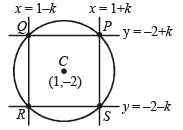Let the side of square be 2k.
Then sides of square are x = 1 – k and x = 1 + k and y = – 2 – k and y = – 2 + k
∴ Co-ordinates of P, Q, R, S are (1 + k, – 2 + k), (1 – k, – 2 + k), (1 – k, – 2 – k), (1 + k, – 2  – k) respectively.
Also P (1 + k, – 2 + k) lies on circle
∴ (1 + k)2 + (– 2 + k)2 – 2 (1 + k) + 4 (–2 + k) + 3 = 0 ⇒ 2k2 = 2
⇒ k = 1  or – 1 If k = 1,  P (2, – 1), Q (0, – 1), R (0, – 3), S (2, – 3)
If k = – 1,  P (0, – 3), Q (2, – 3), R (2, – 1), S(0, – 1)

Test: Single Correct MCQs: Circle | JEE Advanced - Question 2

### Two circles x2 + y2 = 6 and x2 + y2 – 6x + 8 = 0 are given. Then the equation of the circle through their points of intersection and the point (1, 1) is (1980)

Detailed Solution for Test: Single Correct MCQs: Circle | JEE Advanced - Question 2

The circle through points of intersection of the two circles x2 + y2 – 6 = 0
and  x2 + y2 – 6x + 8 = 0
is (x2 + y2 – 6) + l (x2 + y2 – 6x + 8) = 0
As it passes through (1, 1) (1 + 1 – 6) + l (1 + 1 – 6 + 8) = 0  ⇒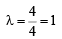∴ The required circle is 2x2 + 2y2 – 6x + 2 = 0
or,    x2 + y2 – 3x + 1 = 0

Test: Single Correct MCQs: Circle | JEE Advanced - Question 3

### The centre of the circle passing through the point (0, 1) and touching the curve y = x2 at (2, 4) is (1983 - 1 Mark)

Detailed Solution for Test: Single Correct MCQs: Circle | JEE Advanced - Question 3

Let C (h, k) be the centre of circle touching x2 = y at B (2, 4). Then equation of common tangent at B is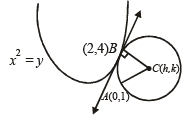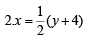i.e., 4x – y = 4

Radius is perpendicular to this tangent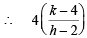= -1 ⇒ 4k = 18… (1)

Also AC = BC ⇒ h2 + (k – 1)2 = (h – 2)2 + (k – 4)2
⇒ 4h + 6k = 19 … (2)

Solving (1) and (2) we get the centre as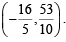Test: Single Correct MCQs: Circle | JEE Advanced - Question 4

The equation of the circle passing through (1, 1) and the points of intersection of x2 + y2 + 13x – 3y = 0 and 2x2 + 2y2 + 4x – 7y – 25 = 0 is (1983 - 1 Mark)

Detailed Solution for Test: Single Correct MCQs: Circle | JEE Advanced - Question 4

KEY CONCEPT
Circle through pts. of intersection of two circles S1 = 0 and S2 = 0 is S1 + λS2 = 0
∴ Req. circle is, (x2 + y2 + 13x – 3y) + λ (x2 + y2 + 2x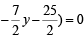⇒ (1 + λ) x2 + (1 +λ) y2 + (13 + 2λ)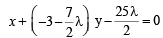As it passes through (1, 1)

∴ 1 + λ + 1 + λ + 13 + 2λ – 3 –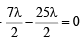⇒ – 12λ + 12 = 0   ⇒ λ = 1

∴ Req. circle is,

2x2 + 2y2 + 15 x -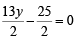or4x2 + 4y2 + 30x –13 y – 25 = 0

Test: Single Correct MCQs: Circle | JEE Advanced - Question 5

The locus of the mid -point of a chord of the circle x2 + y2 = 4 which subtends a right angle at the origin is        (1984 - 2 Marks)

Detailed Solution for Test: Single Correct MCQs: Circle | JEE Advanced - Question 5

Let AB be the chord with its mid pt M (h, k ).

As ∠AOB = 90°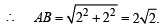∴ AM =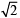NOTE THIS STEP
By prop. of rt. Δ AM = MB = OM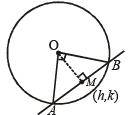∴ OM =⇒ h2 +k= 2

∴ locus of (h, k) is x2 + y2 = 2

Test: Single Correct MCQs: Circle | JEE Advanced - Question 6

If a circle passes through the point (a, b) and cuts the circle x2 + y2 = k2 orthogonally, then the equation of the locus of its centre is (1988 - 2 Marks)

Detailed Solution for Test: Single Correct MCQs: Circle | JEE Advanced - Question 6

KEY CONCEPT
Two circles x2 + y2 + 2g1x + 2f1y + c1 = 0 and
x2 + y2 + 2g2 x + 2f2 y + c2 = 0 are orthogonal iff
2g1g2 + 2f1 f2 = c1 + c2

(a) Let the required circle be, x2 + y2 + 2gx + 2fy + c = 0 … (1)
As it passes through (a, b), we get,
a2 + b2 + 2ag + 2bf + c = 0 … (2)
Also (1) is orthogonal with the circle, x2 + y2 = k2 … (3)
For circle (1) g1 = g, f1 = f, c1 = c
For circle (3) g2 = 0, f2 = 0, c2 = – k2
∴ By the condition of orthogonality,
2g1g2 + 2f1f2 = c1 + c2 We get, c = k2
Substituting this value of c in eq. (2), we get
a2 + b2 + 2ga + 2f b + k2 = 0
∴ Locus of centre (g – f) of the circle can be obtained by replacing g by –x and f  by – y
we get a2 + b2 – 2ax – 2by + k2 = 0
i.e.2ax + 2by – (a2 + b2 + k2) = 0

Test: Single Correct MCQs: Circle | JEE Advanced - Question 7

If the two circles (x – 1)2 + (y – 3)2 = r2 and x2 + y2 – 8x + 2y + 8 = 0 intersect in two distinct points, then (1989 - 2 Marks)

Detailed Solution for Test: Single Correct MCQs: Circle | JEE Advanced - Question 7

We have two circles (x – 1)2 + (y – 3)2 = r2
Centre (1, 3), radius  = r and x2 + y2 – 8x + 2y + 8 = 0

Centre (4, – 1), radius =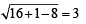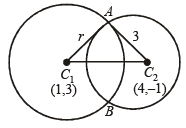As the two circles intersect each other in two distinct points we should have

C1 C2 < r1 + r2 and C1 C2 > | r1 – r2 |

⇒ C1 C2 < r + 3 and C1 C2 < | r1 – r2|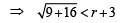and 5 > |r – 3 |

⇒ 5 < r + 3 ⇒ | r – 3 | < 5        ⇒ r > 2 … (i)

⇒ – 5 < r – 3 < 5              ⇒ – 2 < r < 8 … (ii)

Combining (i) and (ii), we get 2 < r < 8

Test: Single Correct MCQs: Circle | JEE Advanced - Question 8

The lines 2x – 3y = 5 and 3x – 4y = 7 are diameters of a circle of area 154 sq. units. Then the equation of this circle is

Detailed Solution for Test: Single Correct MCQs: Circle | JEE Advanced - Question 8

As 2x – 3y – 5 = 0 and 3x – 4y – 7 = 0 are diameters of circles.

∴ Centre of circle is solution of these two eq. ' s, i.e.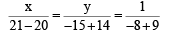⇒ x = 1, y = – 1

∴ C (1, –1)
Also area of circle, πr2 = 154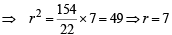∴ Equation of required circle is

(x – 1)2 + (y + 1)2 = 72

⇒ x2 + y2 – 2x + 2y = 47

Test: Single Correct MCQs: Circle | JEE Advanced - Question 9

The centre of a circle passing through the points (0, 0), (1, 0) and touching the circle x2 + y2 = 9 is (1992 -  2 Marks)

Detailed Solution for Test: Single Correct MCQs: Circle | JEE Advanced - Question 9

Let the equation of the circle be
x2 + y2 + 2gx + 2fy + c = 0.
As this circle passes through (0, 0) and (1, 0)
we get c = 0, 1 + 2g = 0

⇒ g =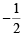According to the question this circle touches the given circle x2 + y2 = 9

∴ 2 × radius of required circle = radius of given circle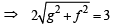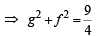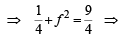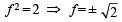∴ The centre is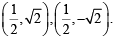Test: Single Correct MCQs: Circle | JEE Advanced - Question 10

The locus of the centre of a circle, which touches externally the circle x2 + y2 – 6x – 6y + 14 = 0 and also touches the y-axis, is given by the equation: (1993 -  1 Marks)

Detailed Solution for Test: Single Correct MCQs: Circle | JEE Advanced - Question 10

The given circle is x2 + y2 – 6x + 14 = 0, centre (3, 3), radius = 2
Let (h, k) be the centre of touching circle.

Then radius of touching circle = h [as it touches y-axis also]

∴ Distance between centres of two circles =  sum of the radii of two circles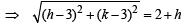⇒ (h – 3)2 + (k – 3)2 = (2 + h)2

⇒ k2 – 10h – 6k + 14 = 0

∴ locus of (h, k) is  y2 – 10x – 6y + 14 = 0

Test: Single Correct MCQs: Circle | JEE Advanced - Question 11

The circles x2 + y2 – 10x + 16 = 0 and x2 + y2 = r2 intersect each other in two distinct points if (1994)

Detailed Solution for Test: Single Correct MCQs: Circle | JEE Advanced - Question 11

Centres and radii of two circles are C1 (5, 0); 3 = r1 and C2 (0, 0); r = r2

As circles intersect each other in two distinct points,

|r1 – r2| < C1C2 < r1 + r2

⇒ | r – 3 | < 5 < r + 3 ⇒ 2 < r < 8

Test: Single Correct MCQs: Circle | JEE Advanced - Question 12

The angle between a pair of tangents drawn from a point P to the circle x2 + y2 + 4x – 6y + 9 sin2α + 13 cos2α = 0 is 2α.The equation of the locus of the point P is (1996 - 1 Mark)

Detailed Solution for Test: Single Correct MCQs: Circle | JEE Advanced - Question 12

Centre of the circle x2 + y2 + 4x – 6y + 9 sin2α + 13 cos2α = 0 is C (– 2, 3) and its radius is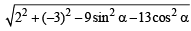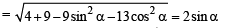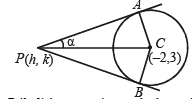Let P (h, k) be any point on the locus. The ∠ APC =α

Also ∠ PAC =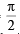That is, triangle APC is a right triangle.

Thus,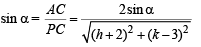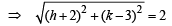⇒ (h + 2)2 + (k – 3)2 = 4 or    h2 + k2 + 4h – 6k + 9 = 0

Thus required equation of the locus is x2 + y2 + 4x – 6y + 9 = 0

Test: Single Correct MCQs: Circle | JEE Advanced - Question 13

If two distinct chords, drawn from the point (p, q) on the circle x2 + y2 = px + qy (where pq ≠ 0) are bisected by the x –axis, then (1999 - 2 Marks)

Detailed Solution for Test: Single Correct MCQs: Circle | JEE Advanced - Question 13

The given equation of the circle is x2 + y2 – px – qy  = 0, pq ≠ 0 Let the chord drawn from (p, q) is bisected by x-axis at point (x1, 0).
Then equation of chord is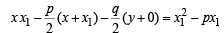(using T = S1)

As it passes through (p, q), therefore,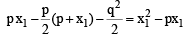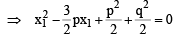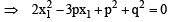As through (p,q) two distinct chords can be drawn.

∴ Roots of above equation be real and distinct,  i.e., D > 0.

⇒ 9p2 – 4 × 2 (p2 + q2) > 0 ⇒ p2 > 8q2

Test: Single Correct MCQs: Circle | JEE Advanced - Question 14

The triangle PQR is inscribed in the circle x2 + y2 = 25. If Q and R have co-ordinates (3,4) and (–4, 3) respectively, then ∠QPR is equal to (2000S)

Detailed Solution for Test: Single Correct MCQs: Circle | JEE Advanced - Question 14

O is the point at centre and P is the point at circumference. Therefore, angle QOR is double the angle QPR.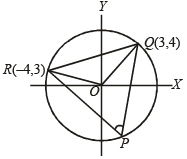So, it sufficient to find the angle QOR. Now slope of OQ = 4/3
Slope of OR = – 3/4
Again m1 m2 = – 1
Therefore, ∠QOR = 90° which implies that ∠QPR = 45°.

Test: Single Correct MCQs: Circle | JEE Advanced - Question 15

If the circles x2 + y2 + 2x + 2ky + 6 = 0, x2 + y2 + 2ky + k = 0 intersect orthogonally, then k is (2000S)

Detailed Solution for Test: Single Correct MCQs: Circle | JEE Advanced - Question 15

2g1g2 + 2f1f2 = c1+ c2 (formula for orthogonal intersection of two cricles)

⇒ 2 (1) (0) + 2 (k) (k) = 6 + k

⇒ 2k2 – k – 6 = 0

⇒ k = – 3/2, 2

Test: Single Correct MCQs: Circle | JEE Advanced - Question 16

Let AB be a chord of the circle x2 + y2 = r2 subtending a right angle at the centre. Then the locus of the centroid of the triangle PAB as P moves on the circle is (2001S)

Detailed Solution for Test: Single Correct MCQs: Circle | JEE Advanced - Question 16

x2 + y2 = r2 is a circle with centre at (0, 0) and radius r units.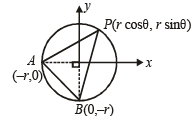Any arbitrary pt P on it is (r cosθ , r sinθ ) Choosing A and B as (– r, 0) and (0, – r).

[So that ∠AOB = 90°]

For locus of centriod of ΔABP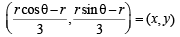⇒ r cosθ – r = 3x
r sinθ – r = 3y

⇒ r cosθ = 3x + r
r sinθ = 3y + r

Squaring and adding (3x + r)2 + (3y + r)2 = r2 which is a circle.

Test: Single Correct MCQs: Circle | JEE Advanced - Question 17

Let PQ and RS be tangents at the extremities of the diameter PR of a circle of radius r. If PS and RQ intersect at a point X on the circumference of the circle, then 2r equals (2001S)

Detailed Solution for Test: Single Correct MCQs: Circle | JEE Advanced - Question 17

Let ∠RPS = θ

∠XPQ = 90° – θ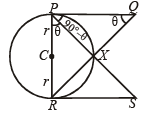∴∠PQX = θ (∵ ∠PXQ = 90°)

∴ΔPRS ~ ΔQPR (AA similarity)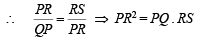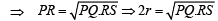Test: Single Correct MCQs: Circle | JEE Advanced - Question 18

If the tangent at the point P on the circle x2 + y2 + 6x + 6y = 2 meets a straight line 5x –2y + 6 = 0 at a point Q on the y - axis, then the length of PQ is (2002S)

Detailed Solution for Test: Single Correct MCQs: Circle | JEE Advanced - Question 18

Line 5x – 2y + 6 = 0 is intersected by tangent at P to circle x2 + y2 + 6x + 6y – 2 = 0 on y-axis at Q (0, 3).
In other words tangent passes through (0, 3)
∴ PQ = length of tangent to circle from (0, 3)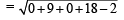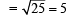Test: Single Correct MCQs: Circle | JEE Advanced - Question 19

The centre of circle inscribed in square formed by the lines x2 – 8x + 12 = 0 and y2 – 14y + 45 = 0, is (2003S)

Detailed Solution for Test: Single Correct MCQs: Circle | JEE Advanced - Question 19

x2 – 8x + 12 = 0 ⇒ (x – 6) (x – 2) = 0
y2 – 14y + 45 = 0 ⇒ (y – 5) (y – 9) = 0
Thus sides of square are x = 2, x = 6, y = 5, y = 9
Then centre of circle inscribed in square will be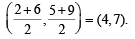Test: Single Correct MCQs: Circle | JEE Advanced - Question 20

If one of the diameters of the circle x2 + y2 – 2x – 6y + 6 = 0 is a chord to the circle with centre (2, 1), then the radius of the circle is (2004S)

Detailed Solution for Test: Single Correct MCQs: Circle | JEE Advanced - Question 20

The given circle is x2 + y2 – 2x – 6y + 6 = 0 with centre C(1, 3) and radius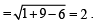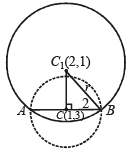Let AB be one of its diameter which is the chord of other circle with centre at C1 (2, 1).
Then in ΔC1CB,r2 = [(2 – 1)2 + (1 – 3)2] + (2)2

⇒ r2 = 1 + 4 + 4  ⇒ r2 = 9  ⇒ r = 3.

Test: Single Correct MCQs: Circle | JEE Advanced - Question 21

A circle is given by x2 + (y–1)2 = 1, another circle C touches it externally and also the x-axis, then the locus of its centre is (2005S)

Detailed Solution for Test: Single Correct MCQs: Circle | JEE Advanced - Question 21

Let the centre of circle C be (h, k). Then as this circle touches axis of x, its radius = | k |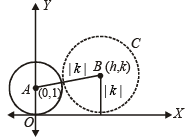Also it touches the given circle x2 + (y – 1)2 = 1, centre (0, 1) radius 1, externally

Therefore, the distance between centres = sum of radii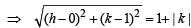⇒ h2 + k2 – 2k + 1 = (1 + | k |)2
⇒ h2 +  k2 – 2k + 1 = 1 + 2 | k | + k2
⇒ h2 = 2k + 2 | k |
∴ Locus of (h, k) is, x2 = 2y + 2 | y |
Now if y > 0, it becomes x2 = 4y and if y < 0, it becomes x = 0
∴ Combining the two, the required locus is {(x, y) : x2 = 4y} ∪ {(0, y) : y < 0}

Test: Single Correct MCQs: Circle | JEE Advanced - Question 22

Tangents drawn from the point P(1, 8) to the circle x2 + y2 –6x– 4y –11 = 0 touch the circle at the points A and B. The equation of the circumcircle of the triangle PAB is (2009)

Detailed Solution for Test: Single Correct MCQs: Circle | JEE Advanced - Question 22

Tangents PA and PB are drawn from the point P (1, 3) to circle x2 + y2 - 6 x - 4y - 11=0 with centre C (3, 2)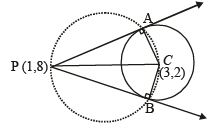Clearly the circumcircle of ΔPAB will pass through C and as ∠A = 90°, PC must be a diameter of the circle.

∴ Equation of required circle is (x -1)(x - 3) + (y-8)(y - 2)= 0

⇒ x2 + y2 - 4x - 10y + 19= 0

Test: Single Correct MCQs: Circle | JEE Advanced - Question 23

The circle passing through the point (– 1, 0) and touching the y-axis at (0, 2) also passes through the point.     (2011)

Detailed Solution for Test: Single Correct MCQs: Circle | JEE Advanced - Question 23

Let centre of the circle be (h, 2) then radius = |h|

∴ Equation of circle becomes (x – h)2 + (y – 2)2 = h2

As it passes through (–1, 0)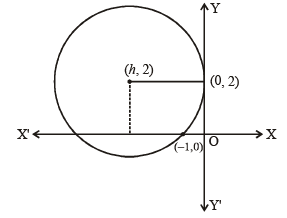⇒ (–1 – h)2 + 4 = h2 ⇒ h =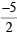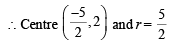Distance of centre from (–4, 0) is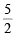∴ It lies on the circle.

Test: Single Correct MCQs: Circle | JEE Advanced - Question 24

The locus of th e mid-point of the ch or d of con tact of tangents drawn from points lying on the straight line 4x – 5y = 20 to the circle x2 + y2 = 9 is     (2012)

Detailed Solution for Test: Single Correct MCQs: Circle | JEE Advanced - Question 24

Any point P on line  4x – 5y = 20  is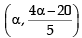Equation of chord of contact AB to the circle x2 + y= 9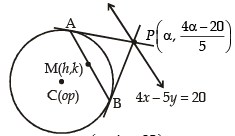drawn from point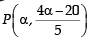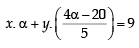....(1)

Also the equation of chord AB whose mid point is (h, k) is

hx + ky = h2+k2 ....(2)

∵  Equations (1) and (2) represent the same line, therefore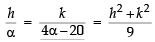⇒ 5kα= 4hα- 20h and 9h = α( h2+k2)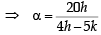and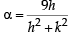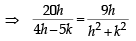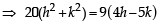∴ Locus of (h, k) is

(20 x2 + y2) - 36x + 45y= 0

## Maths 35 Years JEE Main & Advanced Past year Papers

132 docs|70 tests
 Use Code STAYHOME200 and get INR 200 additional OFF Use Coupon Code